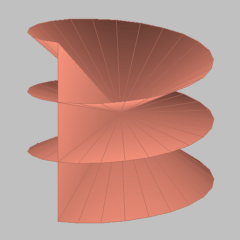## Riemann SurfacesElectronic Geometry Model No. 2002.05.001

#### Description

Riemann surfaces were first studied by Bernhard Riemann in his Inauguraldissertation at Göttingen in 1851.

Consider the function from the complex plane to itself given by w=f(z)=zn, where n is at least 2. The z-plane may be divided into n sectors given by arg z lying between (k-1)(2pi/n) and k(2pi/n) for k=1,...,n. There is a one-to-one correspondence between each sector and the whole w-plane, except for the positive real axis. The image of each sector is obtained by performing a cut along the positive real axis; this cut has an upper and a lower edge. Corresponding to the n sectors in the z-plane, take n identical copies of the w-plane with the cut. These will be the sheets of the Riemann surface and are distinguished by a label k which serves to identify the corresponding sector. For k=1,...,n-1 attach the lower edge of the sheet labeled k with the upper edge of the sheet labeled k+1. To complete the cycle, attach the lower edge of the sheet labeled n to the upper edge of the sheet labeled 1. In a physical sense, this is not possible without self-intersection but the idealized model shall be free of this discrepancy. The result of the construction is a Riemann surface whose points are in one-to-one correspondence with the points of the z-plane. This correspondence is continuous in the following sense. When z moves in its plane the corresponding point w is free to move on the Riemann surface. The point w=0 connects all the sheets and is called the branch point. A curve must wind n times around the branch point before it closes. Now consider the n-valued relation z=nthroot(w). To each nonzero w, there correspond n values of z. If the w-plane is replaced by the Riemann surface just constructed, then each complex nonzero w is represented by n points of the Riemann surface at superposed positions. Let the point on the uppermost sheet represent the principal value and the other n-1 points represent the other values. Then z=nthroot(w) becomes a single-valued, continuous, one-to-one correspondence of the points of the Riemann surface with the points of the z-plane. The Riemann surface is orientable, since every orientation of a sheet is carried over to the sheet next to it.

Model produced with: JavaView v.2.21

 Keywords Riemann Surface; Algebraic Function MSC-2000 Classification 30F99 Zentralblatt No. 05264891

#### References

1. B. Riemann: Grundlagen für eine allgemeine Theorie der Functionen einer veränderlichen complexen Grösse, Inauguraldissertation, Göttingen (1851), http://www.emis.de/classics/Riemann/.
2. D. Hilbert and S. Cohn-Vossen: Anschauliche Geometrie, English Translation by Chelsea Publishing Company (1990) (1932), .

#### Submission information

Submitted: Sun May 19 09:03:19 CEST 2002.
Revised: Fri Jan 10 14:19:31 GMT 2003.
Accepted: Fri Feb 14 18:02:36 CET 2003.